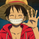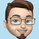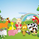# Moving Average Delta Indicator by KIVANC fr3762

3553 görüntülenme
3553
Description:
MAD stands for Moving Average Delta, it calculates the difference between moving average and price. The curve shows the difference in Pips.

By calculating the delta between two points we can see more small changes in the direction of the moving average curve which are normally hard to see. You can see the MAD curve as look through the microscope at a simple moving average curve. It may help predicting a trend change before it happens, the sample shows a beginning trend change from long to short.

Interpretation:

If the MAD curve is bigger than 0, the moving average is above the price
conversely;
If the MAD curve is smaller than 0, the moving average is below the price

Before a trend change, the moving average gets flatter, the MAD curve points to towards the zero
We can see what is the maximum rising/falling of the difference and predict an upcomming trend change
Usage:

Drop a simple moving average to a chart and set the period in a way that it best fits the movements. There is no "magic" settings for the moving average period, you may double click the MA line to set it to a different period.

Drop the MAD indicator to the cart and give it the same period as your simple moving average .